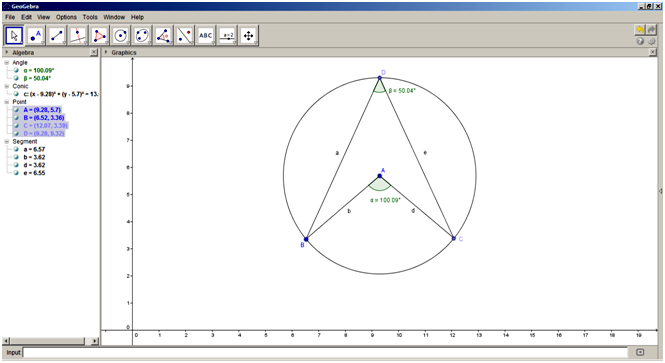## ICT PRACTICALS

### P10

SSC BOARD EXAM ICT Practical P10 - Verify the theorem using Geogebra.

Practical Name: Verify the theorem using Geogebra - The measure of an angle subtended by an arc at a point on the circle is half of the measure of the angle subtended by the same arc at the centre.

Tools/Software: Computer with Geogebra Software installed.

Procedure:

1)          Start the Geogebra Software
2)         Select the Circle with Centre through Point tool. Click at a point A and then click at another convenient point B to draw a circle with centre A through point B.
3)         Using New Point tool, add two other points C and D on the circumference of the circle
4)         Select the Segment between Two Points tool and draw the segments AB, AC, DB and DC.
5)         Select the Angle tool. Click on the segments AB and AC. This measures the angle subtended by the arc CB at the centre as α. Similarly, click on the segments DB and DC. This measures the angle subtended by the arc CB at the circumference as β.
6)         It is seen that α=2β, i.e. the measure of the angle subtended by an arc at the circumference is half that subtended at the centre.

Result: Theorem verified.#### 1 comment:

1.Great article and helpful and I have seen same Blog at GK EXAM please tell me which one of best Blog for GK Book in Hindi??